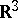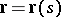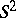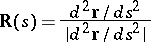# Spherical indicatrix

(diff) ← Older revision | Latest revision (diff) | Newer revision → (diff)

The image of a curve in the three-dimensional Euclidean spaceunder a mapping from the points of the curve onto the unit sphereby any of the following unit vectors: the tangent, the principal normal or the binormal of this curve. Letbe the radius vector of the curve, letbe the natural parameter and letbe the radius vector of the spherical mapping of the curveinto the unit spherewith its centre at the origin by means of one of the unit vectors listed. The equation of the spherical indicatrix of the tangent is defined by the equationthat of the spherical indicatrix of the principal normal by the equationand that of the spherical indicatrix of the binormal by the equationThe tangent to the spherical indicatrix of the tangent is parallel to the principal normal of the curve at the corresponding values. The curvature and the torsion of the spherical indicatrix can be expressed in terms of the curvature and torsion of the curve itself. For every spherical indicatrix there is an infinite set of curves for which it is an indicatrix, i.e. a curve cannot be uniquely restored from its spherical indicatrix.

How to Cite This Entry:
Spherical indicatrix. Encyclopedia of Mathematics. URL: http://encyclopediaofmath.org/index.php?title=Spherical_indicatrix&oldid=17468
This article was adapted from an original article by L.A. Sidorov (originator), which appeared in Encyclopedia of Mathematics - ISBN 1402006098. See original article